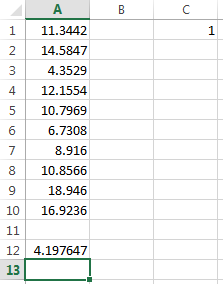# matlabfcn

Evaluate MATLAB command given Microsoft Excel data

## Syntax

``= matlabfcn(command,inputs)``

## Description

example

````= matlabfcn(command,inputs)` specifies the MATLAB® command for evaluation in the MATLAB workspace, given the input data `inputs`. Depending upon the MATLAB output, `matlabfcn` returns a single value or string into the calling worksheet cell. If the result contains more than one value in MATLAB, only the first value in the array returns in the calling worksheet cell. Use this syntax when working in a worksheet cell.```

## Examples

collapse all

Using `std`, find the maximum likelihood estimate of the standard deviation of data in worksheet cells `A1` through `A10`. Assume that the data comes from a normal population. Specify a weight of one in cell `C1`. Return the result by entering this text into cell `A12`.

`=matlabfcn("std",A1:A10,C1)`Cell `A12` displays the maximum likelihood estimate of the standard deviation.## Input Arguments

collapse all

MATLAB command to evaluate, specified as a string. Enclose the string in double quotes. Or, enter the string in a cell without quotes and enter the corresponding cell reference without quotes as the input argument.

Example: `"sum"`

Example: `A1`

MATLAB command input arguments, specified as an Excel cell reference or a reference to a range of cells. To specify multiple input arguments for a function, separate the cell references with commas.

Example: `B1:B10`

Example: `A1:C1,A3`

## Tips

• If `matlabfcn` fails, a standard Spreadsheet Link™ error displays by default; for example, `#COMMAND`. To return MATLAB errors, use `MLShowMatlabErrors`.

• To execute a MATLAB command that returns no data, use the `MLEvalString` function instead of `matlabfcn`. In this case, write a command such as `MLEvalString("function(" & A1 & ")")`, where `function` is the name of the function that returns no data and `A1` is an input argument.

## Version History

Introduced before R2006a